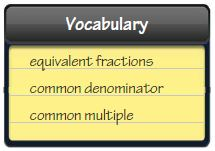Homework Explained - Math Practice 101Dear guest, you are not a registered member. As a guest, you only have read-only access to our books, tests and other practice materials.

As a registered member you can:

Registration is free and doesn't require any type of payment information. Click here to Register.
Go to page:
Grade 5 HMH Go Math - NEW
Chapter 6 Mid-Chapter Checkpoint

## Vocabulary

Choose the best term from the box.• Question 1

A ________ is a number that is a multiple of two or more numbers.

• Question 2

A ________ is a common multiple of two or more denominators.

## Concepts and Skills

Estimate the sum or difference.

• Question 3

$$\frac{8}{9}+\frac{4}{7}$$

• Question 4

$$3\cfrac{2}{5}-\cfrac{5}{8}$$

• Question 5

$$1\frac{5}{6}+2\frac{2}{11}$$

Use a common denominator to write an equivalent fraction for each fraction.

• Question 6

$$\frac{1}{6}, \frac{1}{9}$$

common denominator:

• Type below:
• Question 7

$$\frac{3}{8}, \frac{3}{10}$$

common denominator:

• Type below:
• Question 8

$$\frac{1}{9}, \frac{5}{12}$$

common denominator:

• Type below:

Use the least common denominator to write an equivalent fraction for each fraction.

• Question 9

$$\frac{2}{5}, \frac{1}{10}$$

• least common denominator:
• Explain:
• Question 10

$$\frac{5}{6}, \frac{3}{8}$$

• least common denominator:
• Explain:
• Question 11

$$\frac{1}{3}, \frac{2}{7}$$

• least common denominator:
• Explain:

• Question 12

$$\frac{11}{18} - \frac{1}{6}$$

• Question 13

$$\frac{2}{7} + \frac{2}{5}$$

• Question 14

$$\frac{3}{4} - \frac{3}{10}$$

Yes, email page to my online tutor. (if you didn't add a tutor yet, you can add one here)